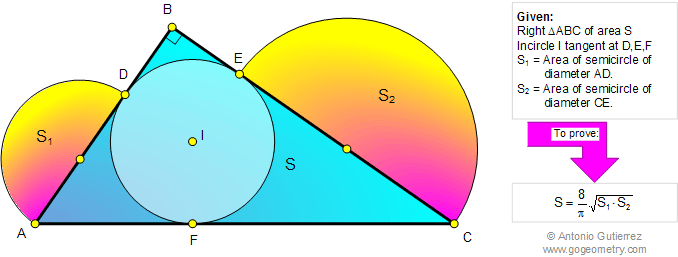# Online Geometry Problem 807: Right Triangle Area, Incircle, Tangency Points, Semicircle Area, Diameter. Level: High School, SAT Prep, College, Mathematics Education

 The figure below shows a right triangle ABC of area S with the incircle I tangent to AB, BC, and AC at D, E, and F, respectively. If S1 and S2 are the areas of semicircles of diameters AD and CE, prove that.Geometry problem solving is one of the most challenging skills for students to learn. When a problem requires auxiliary construction, the difficulty of the problem increases drastically, perhaps because deciding which construction to make is an ill-structured problem. By “construction,” we mean adding geometric figures (points, lines, planes) to a problem figure that wasn’t mentioned as "given.
 Home | Search | Geometry | Problems | All Problems | Open Problems | Visual Index | 10 Problems | 801-810 | Triangles | Incircle | Circles | Right Triangle Area | Semicircle | Email | Solution / comment | By Antonio Gutierrez